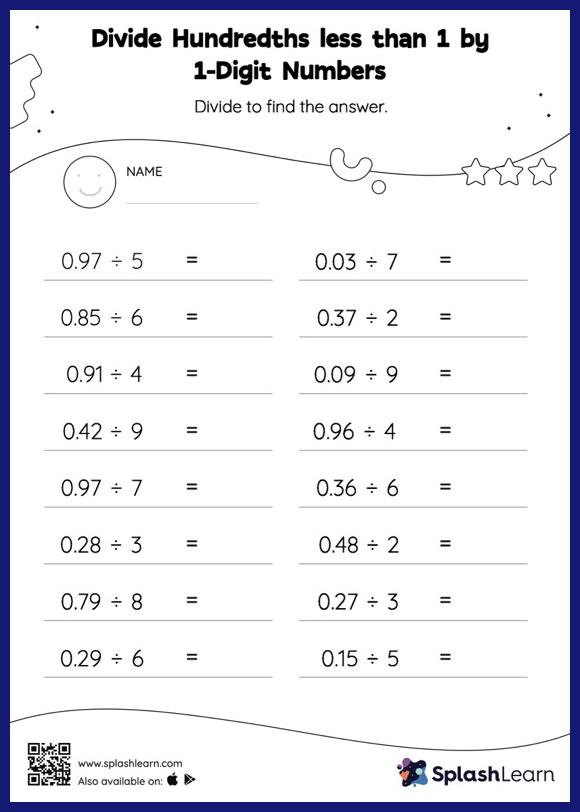# Divide Hundredths less than 1 by 1-Digit Numbers: Horizontal Division Worksheet

Home > Divide Hundredths less than 1 by 1-Digit Numbers: Horizontal DivisionChildren practice dividing hundredths less than 1 by 1-digit numbers with this worksheet. Students begin by dividing decimals by 1-digit integers in the same manner they would with whole numbers. Depending on the number of decimal digits in the dividend, they add a decimal point at the appropriate location in the quotient. They get more confidence with this concept thanks to divide hundredths less than 1 by 1-digit numbers worksheet.